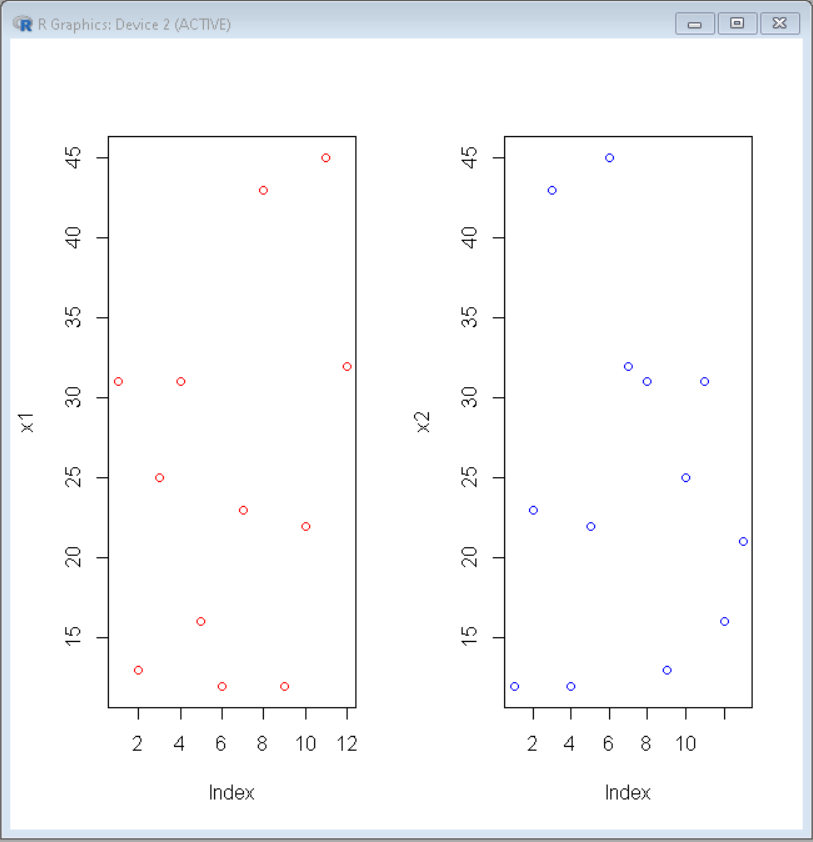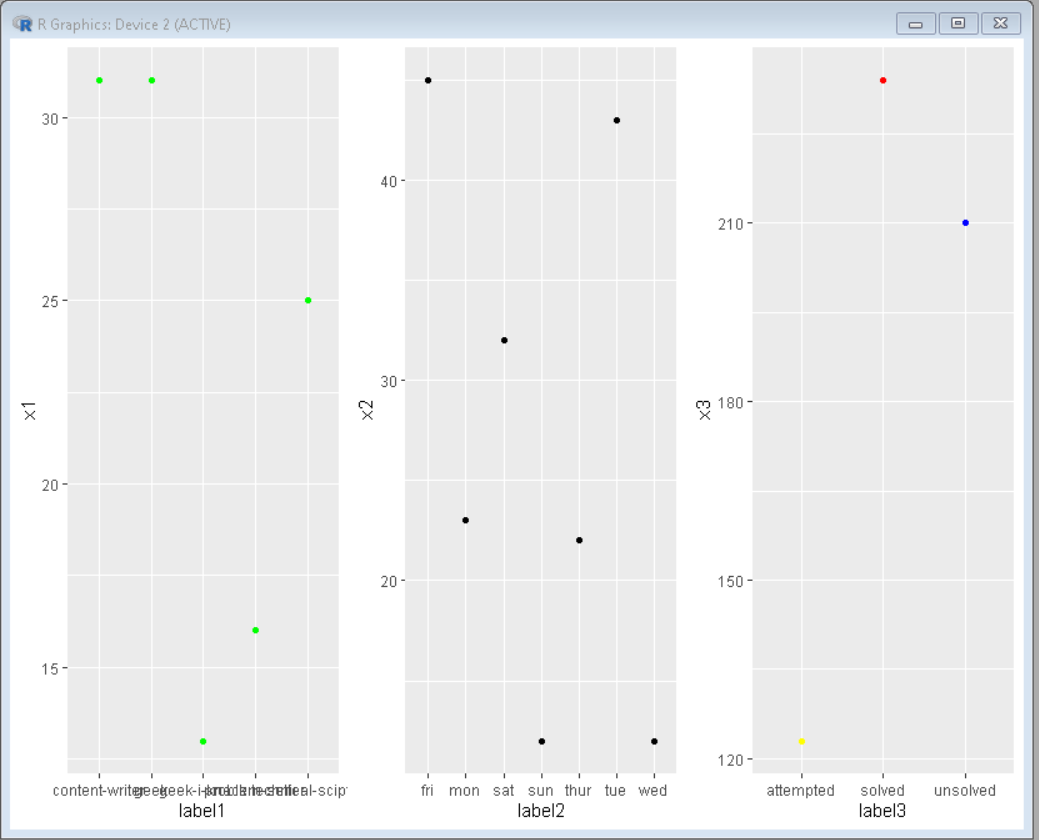Side by Side Scatter charts in R

• Last Updated : 21 Jul, 2021

In this article, we will discuss how to make side by side scatter plot in R Programming Language.

Method 1: Using par() function

Here we will use par() function to divide frames into grids.

Syntax: par(mfrow, mar, mgp, las)

Parameters:

• mfrow: A numeric vector of length 2, which sets the rows and column in which frame has to be divided.
• mar: A numeric vector of length 4, which sets the margin sizes in the following order: bottom, left, top, and right.
• mgp: A numeric vector of length 3, which sets the axis label locations relative to the edge of the inner plot window.
• las: A numeric value indicating the orientation of the tick mark labels and any other text added to a plot after its initialization.

Here, by changing row and column values, we can draw graphs in them. For drawing side-by-side plots we will keep row as 1 and column as the no. of plots required.

R

 # Define data-set columns x1 <- c(31,13,25,31,16,12,23,43,12,22,45,32) x2 <- c(12,23,43,12,22,45,32,31,13,25,31,16,21)     # set the plotting area into a 1*2 array par(mfrow = c(1,2))        # Draw the two scatter chart using above datasets plot(x1, col="red") plot(x2, col="blue")

Output:Method 2: Using ggplot2

Here we will use gridExtra contains a function called arrange() that is used to arrange plots as desired.

Syntax: grid.arrange(plot, nrow, ncol)

Parameter:

• plot: ggplot2 plot which we want to arrange
• nrow: Number of rows
• ncol: Number of columns

Here, by changing nrow and ncol values, we can draw graphs in them. For drawing side-by-side plots we will keep nrow as 1 and ncol as the no. of plots required.

R

 # Define data-set columns x1 <- c(31,13,25,31,16) x2 <- c(12,23,43,12,22,45,32)  x3 <- c(234,123,210)    label1 <- c('geek','geek-i-knack',             'technical-scipter',             'content-writer',             'problem-setter')    label2 <- c('sun','mon','tue',             'wed','thur','fri','sat')    label3 <- c('solved','attempted','unsolved')    # Create data frame using above data column data1 <- data.frame(x1,label1) data2 <- data.frame(x2,label2) data3 <- data.frame(x3,label3)    # set the plotting area into a 1*3 array par(mfrow = c(1,3))    # import library ggplot2 and gridExtra library(ggplot2) library(gridExtra)    # Draw the three scatter chart using above datasets plot1<-ggplot(data1, aes(x=label1, y=x1, group=1)) + geom_point(color="green")    plot2<-ggplot(data2, aes(x=label2, y=x2, group=1)) + geom_point()    plot3<-ggplot(data3, aes(x=label3, y=x3, group=1)) + geom_point(color=c("red","yellow","blue"))    # Use grid.arrange to put plots in columns grid.arrange(plot1, plot2, plot3, ncol=3)

Output:My Personal Notes arrow_drop_up
Recommended Articles
Page :